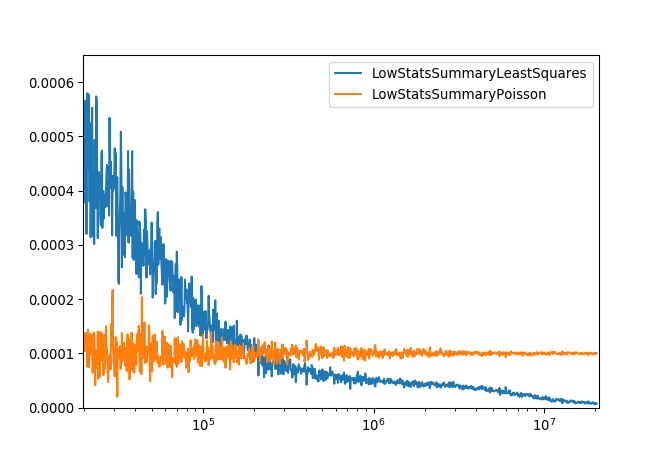$$\renewcommand\AA{\unicode{x212B}}$$

# Poisson Cost Function¶

## Description¶

The Poisson cost function is designed to be applied to data, which has a low number of counts/events. In this scenario a fit is being performed using a model (function) to some low stats data.

The number of counts/events in bin $$i$$ is given by $$y_i$$ and the corresponding value for the model is $$\mu_i$$. Where the values of $$y_i$$ and $$\mu_i$$ are strictly positive.

The quality of the fit can be represented numerically by the deviance ($$D$$)

$2\sum_{i}^{N} \left\{ y_{i} \log(y_i) -y_{i} \log(\mu_i) - (y_{i} - \mu_i) \right\},$

where the first two terms provide a measure for small variations between $$y_i$$ and $$\mu_i$$, whereas the third term provides a measure of large variations. Therefore, by minimizing the deviance the best fit can be identified. This method is also known as the Poisson deviance or Poisson log-linear model.

When performing a fit on Poisson distributed data care needs to be taken when selecting the cost function. For a large number of events/counts the least squares cost function can be used to minimize Poisson distributed data. This is because in the limit of large numbers of events/counts the Poisson and Gaussian distributions are approximately equal. In the limits of low events/counts using a least squares cost function can lead to biased results.

## Example¶

Given a workspace with low counts, a fit can be done in a script as follows: Fit(Function=f,InputWorkspace=workspace,Output="outputName",CreateOutput=True,CostFunction="Poisson",Minimizer="Levenberg-MarquardtMD")

The plot below demonstrates the difference between using the Poisson and Least Squares cost functions for a given parameter over a series of fits to data with different number of counts. The Poisson model gives a much more consistent result and means no special treatment is required for zero counts.